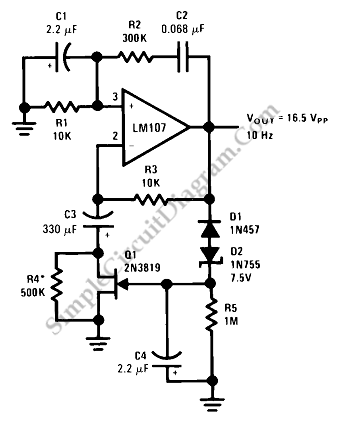Sine Wave Oscillator

This is a Sine Wave Oscillator circuit. This circuit as known as  amplitude-stabilized sine-wave oscillator. It can gives high purity sine-wave output down to low frequencies with minimum circuit complexity. This circuit has a several advantages such as it does not use the traditional tungsten filament lamp amplitude regulator, so the problem problems associated with a lamp such  as linearity and time constant problems are eliminated. besides that, this circuit provides optimum values for a general purpose oscillator. This circuit uses The Wien Bridge topology that is widely used in many applications. Here is the schematic diagram of the circuit:The phase of the applied voltage across the two networks at one particular frequency and that the phase lags with increasing frequency and leads with decreasing frequency is the same with the phase of the voltage across the parallel branch of a series and a parallel RC network connected in series in Wien Bridge topology. Oscillation occurs at the frequency at which the phase shift is zero, when the Wien Bridge used as a positive feedback element around an amplifier. This circuit also has negative feedback that is used to stabilize the frequency of oscillation, to reduce harmonic distortion and to set loop gain to unity at the oscillation frequency.

D1 and D2 are conducted due to negative peaks in excess of ?8.25V and then the C4 is charging. Q1 receives the bias from the charge stored in C4 that determines amplifier gain. This circuit uses low frequency roll-off capacitor, C3, in the feedback network and prevents offset current and offset voltage errors from being multiplied by amplifier gain. The response time of the negative feedback loop filter, C4 and R5, and amplifier open-loop gain determine the Distortion of this circuit. The FET is operated at a small negative gate bias by adjusting the negative feedback loop using C4. In determining amplitude stabilization oscillator distortion and time constant, we need A trade-off. [Source: National Semiconductor Application Note]